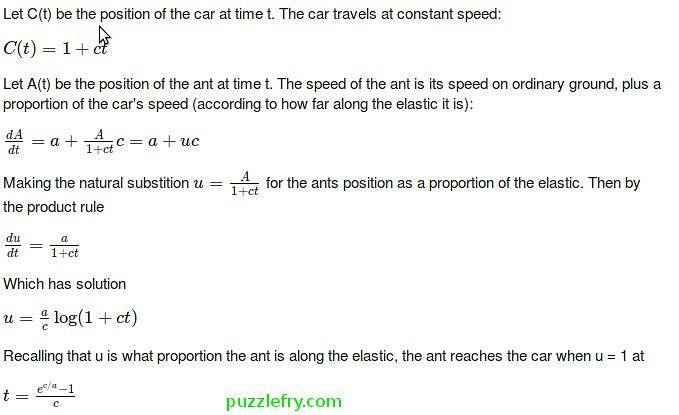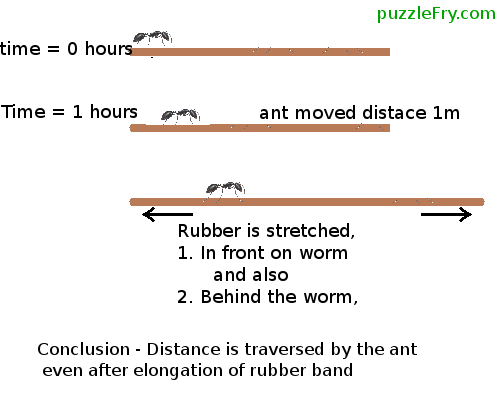SherlockHolmes's Profile
Expert
5361
points

Questions
1441

204

•Expert Asked on 13th August 2015 in

ITERATIVE SOLUTION-

/*
* File:   main.cpp
* Author: akash
*
* Created on 12 August, 2015, 4:57 PM
*/
#include <stdio.h>
#include <stdlib.h>
struct node
{
int data;
struct node* next;
};
//void push(struct **node top_head,int dataToEnter);
//int getCount(struct *node top_head);
void push(struct node** top_head,int dataToEnter)
{
struct node* add_node = (struct node*) malloc(sizeof(struct node));
}
int getCount(struct node* top_head){
if (top_head == NULL)
return 0;
// count is 1 + count of remaining list
return 1 + getCount(top_head->next);
}
int main(){
struct node* head = NULL;
printf("Nodes Count is %d", getCount(head));
return 0;
}

This answer accepted by SherlockHolmes. on 6th November 2015 Earned 20 points.

• 5214 views
•Expert Asked on 11th August 2015 in

The letter “E”.

considering word “week”, it has two similar letters that is “E”
and for the word year, has one “E”.

This answer accepted by SherlockHolmes. on 14th September 2016 Earned 20 points.

• 2035 views
•Expert Asked on 11th August 2015 in

1   2    3

SUM            = 1+2+3=6
PRODUCT = 1*2*3 =6

• 1769 views
•Expert Asked on 10th August 2015 in

Four Coins

Three coins placed flat on the table in a triangle(touching each other) and put the fourth one on top of them in the middle.

• 2696 views
•Expert Asked on 8th August 2015 in

Pi  +  Rats  +  Caribbean

Pirates of the Caribbean

• 3702 views
•Expert Asked on 8th August 2015 in

Take any 13 cards and flip them all. Rest 39 cards will make another pile. These two piles will have the same number of cards facing up. Why? Well, say out of those 13 selected$0 \leq n \leq 13$ cards are facing up.  Which means that in the pile with 39 cards there will be$13-n$ cards facing up. When you flip all 13 cards, number of cards facing up now becomes$13-n$ which is equal to what the other pile have.

• 4633 views
•Expert Asked on 8th August 2015 in

MATHEMATICAL APPROACH-

Yes, the ant will reach the car. Calculus tells us exactly when:if a < 0, then exp(c/a) < 1 and t < 0, which means no solution. The faster the car is, the greater [exp(c/a)]/c is and thus, the longer it is for the ant to reach the car.

• 9502 views
•Expert Asked on 7th August 2015 in

This is Ant on a rubber rope problem

The worm will eventually reach the end.
The key to understand this is that the proportion between the total length of the  rubber Band and the length walked by the worn can only increase with time, as it will eventually approach one.

EXPLAINATION-
The problem seems impossible because we are looking worm and the rubber Band moving independently.

Things become a little more imaginable – “When we realize that the Worm is on the rubber Band, and the portion of the rubber Band behind the worm also stretches just as the portion in front of the worm does,”

The mathematics are complex, but think of the entire picture of the worm and the rubber Band.

At 0 Hour, the worm is at the very end of the rubber Band, and has 100 percent of the rubber Band in front of it.
At 1 Hour, it’s true that the Worms’s task is considerably increased, but it doesn’t have one hundred percent of the rubber Band in front of it.
And that tiny percentage of the rubber Band that the anworm has traversed will stretch in proportion, just like the rest of the rubber Band.

So, Percentage of distance to be covered is increasing in same proportion to the Distance already covered.
i.e.

Percent of Distance traveled is increasing     DIRECTLY PROPORTIONAL to        Increase in distance to be traveled.• 9502 views
•Expert Asked on 7th August 2015 in

It is pretty interesting. Not only can it tell us whether the number is divisible by 7 but also the exact remainder obtained if the number is divided by 7.

How this works is that each node is connected to a remainder by 7. That is each node has a unique number in [0..6] associated with it. And the rest is simple modular arithmetic.

To enumerate the associated numbers, the bottom-most node has 0 associated with it. From there, keep following the black arrows- you’ll obtain the node associated with the next number. As in the right-most node is associated with 1, the topmost with 2 and so on. You’ll notice that the leftmost node is associated with 6 which in turn leads to 0.

You should also notice that the white arrows lead up from nodes n mod 7 to nodes n10 mod 7. This is important.

Now let us consider a number N with digits N1,N2...Nm with Nm being the unit digit.

We begin applying the given algorithm:

1. We follow the black arrow N1 number of times and arrive at the node N1 mod 7.
2. Next we follow the white arrow to arrive at the node N110 mod 7.
3. We repeat step 1 to follow the black arrow N2 number of times. Now we are at N110+N2 mod 7.
4. We follow step 2 again to arrive at N1100+N210 mod 7.
5. Applying the same procedure until the last digit, we finally arrive at the node N110m1+N210m2+..+Nm mod 7 which equals N mod 7. If we are at the bottommost node, then we conclude that N mod 7=0 and hence proving the divisibility of N by 7.

If anything is unclear, feel free to leave comments.

• 4116 views
•Expert Asked on 7th August 2015 in